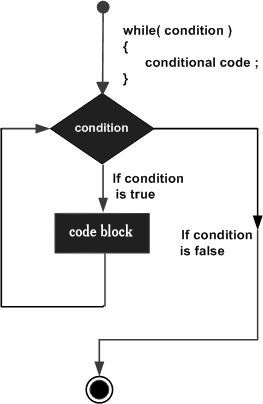# Perl while Loop

A while loop statement in Perl programming language repeatedly executes a target statement as long as a given condition is true.

## Syntax

The syntax of a while loop in Perl programming language is −

```while(condition) {
statement(s);
}
```

Here statement(s) may be a single statement or a block of statements. The condition may be any expression. The loop iterates while the condition is true. When the condition becomes false, program control passes to the line immediately following the loop.

The number 0, the strings '0' and "" , the empty list () , and undef are all false in a boolean context and all other values are true. Negation of a true value by ! or not returns a special false value.

## Flow DiagramHere the key point of the while loop is that the loop might not ever run. When the condition is tested and the result is false, the loop body will be skipped and the first statement after the while loop will be executed.

## Example

```#!/usr/local/bin/perl

\$a = 10;

# while loop execution
while( \$a < 20 ) {
printf "Value of a: \$a\n";
\$a = \$a + 1;
}
```

Here we are using the comparison operator < to compare value of variable \$a against 20. So while value of \$a is less than 20, while loop continues executing a block of code next to it and as soon as the value of \$a becomes equal to 20, it comes out. When executed, above code produces the following result −

```Value of a: 10
Value of a: 11
Value of a: 12
Value of a: 13
Value of a: 14
Value of a: 15
Value of a: 16
Value of a: 17
Value of a: 18
Value of a: 19
```
perl_loops.htm